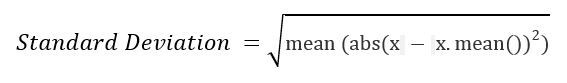# 【整理】numpy mean std

Mean 651浏览
NLP: Text Data Cleaning and Preprocessing – Maria Dobko – Medium
numpy mean std
numpy.std() in Python – GeeksforGeeks
“numpy.std() in Python
numpy.std(arr, axis = None) : Compute the standard deviation of the given data (array elements) along the specified axis(if any).. Standard Deviation (SD) is measured as the spread of data distribution in the given data set.
Standard Deviation (SD)
numpy.mean — NumPy v1.13 Manual
“numpy.std
numpy.std(a, axis=None, dtype=None, out=None, ddof=0, keepdims=<no value>)[source]
Compute the standard deviation along the specified axis.
Returns the standard deviation, a measure of the spread of a distribution, of the array elements. The standard deviation is computed for the flattened array by default, otherwise over the specified axis.”
【总结】

• the spread of a distribution
• 数据分布的广泛程度
• 是否是否很集中
• 方差越大：越分散
• 方差越小：越集中
• numpy的api接口名称：numpy.std

`std = sqrt(mean(abs(x - x.mean())**2))````x = 1 1 1 1 1
Standard Deviation = 0 .

y = 9, 2, 5, 4, 12, 7, 8, 11, 9, 3, 7, 4, 12, 5, 4, 10, 9, 6, 9, 4
Step 1 : Mean of distribution 4 = 7
Step 2 : Summation of (x - x.mean())**2 = 178
Step 3 : Finding Mean = 178 /20 = 8.9
This Result is Variance.
Step 4 : Standard Deviation = sqrt(Variance) = sqrt(8.9) = 2.983..```

91 queries in 0.113 seconds, using 20.49MB memory